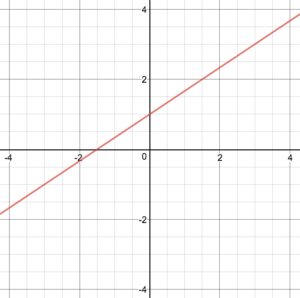## What you’ll learn to do: Graph, write, and analyze equations of linear functions

We can now describe a variety of characteristics that explain the behavior of linear functions. We will use this information to analyze a graphed line and write an equation based on its observable properties. From evaluating the graph, what can you determine about this linear function?• initial value (y-intercept)?
• one or two points?
• slope?
• increasing or decreasing?
• vertical or horizontal?

In this section, you will practice writing linear function equations using the information you’ve gathered. We will also practice graphing linear functions using different methods and predict how the graphs of linear functions will change when parts of the equation are altered.

## Contribute!

Did you have an idea for improving this content? We’d love your input.